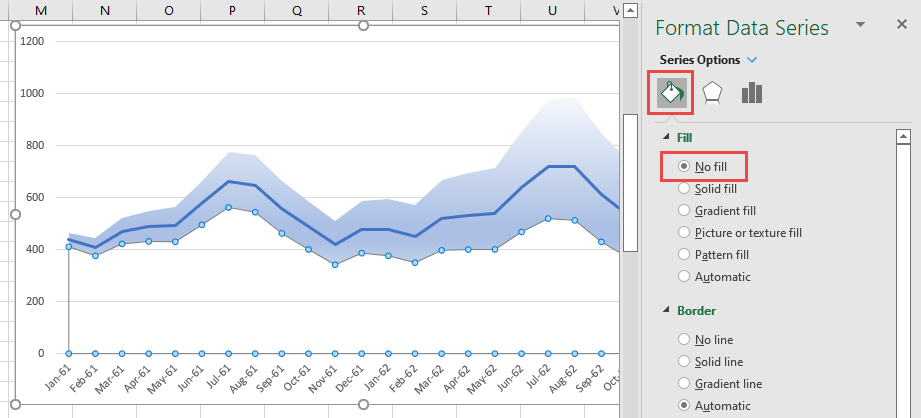# X13ARIMA-SEATS Modeling - Forecasting

This tutorial will outline the steps for setting up the X13ARIMA-SEATS model to generate forecasting values and confidence intervals.

For our sample data, we’ll use the monthly flow of international airline passengers between January 1949 and December 1960. This dataset is a popular time series sample first covered by Box-Jenkins in their time-series reference manual back in 1970.

## Step 1 – Data Preparation

We arranged the observations of the sample data in two adjacent columns: dates and values, in ascending chronological order, so that the first data point corresponds to the earliest date (i.e., Jan 1949) and the last data point corresponds to the latest (i.e., Dec 1960).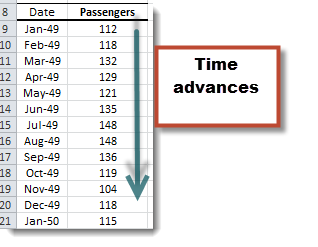IMPORTANT:

We stored the values of the date component as an actual Excel date-type (i.e., date serial number), not text. We chose the display in the “M-YY” date format in the figure above, but you can select any other date format.

Although the X13ARIMA-SEATS model accepts datasets in reverse chronological order (i.e., descending), we chose the ascending order for our demonstration here.

## Step 2 – Define an X13ARIMA-SEATS Model

1. Select an empty cell in your worksheet, preferably one that is close to the input data set and that has a free adjacent cell (to store the model specifications).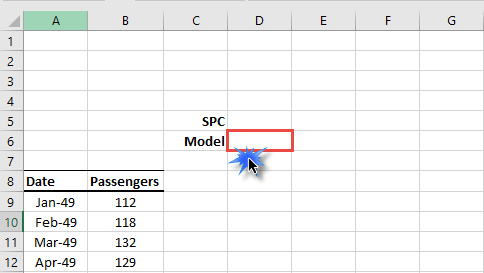Note:

If you already have an X13AS model that you wish to use, select the cell in your worksheet corresponding to that model.

2. From the NumXL toolbar, locate the ARMA icon, click on it, and select the U.S. Census X13ARIMA-SEATS from the drop-down menu.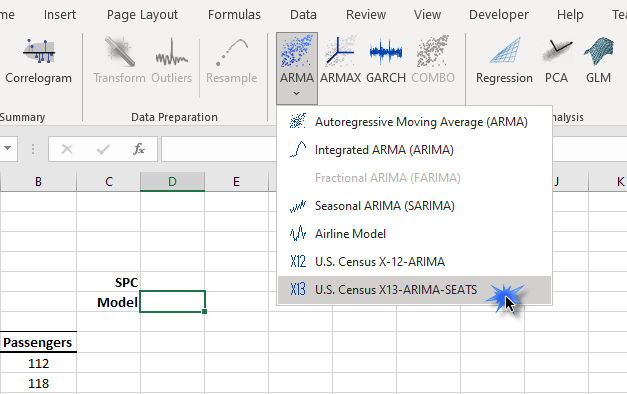3. The X13ARIMA-SEATS Model (aka., X13AS) wizard or dialog box pops up on the screen.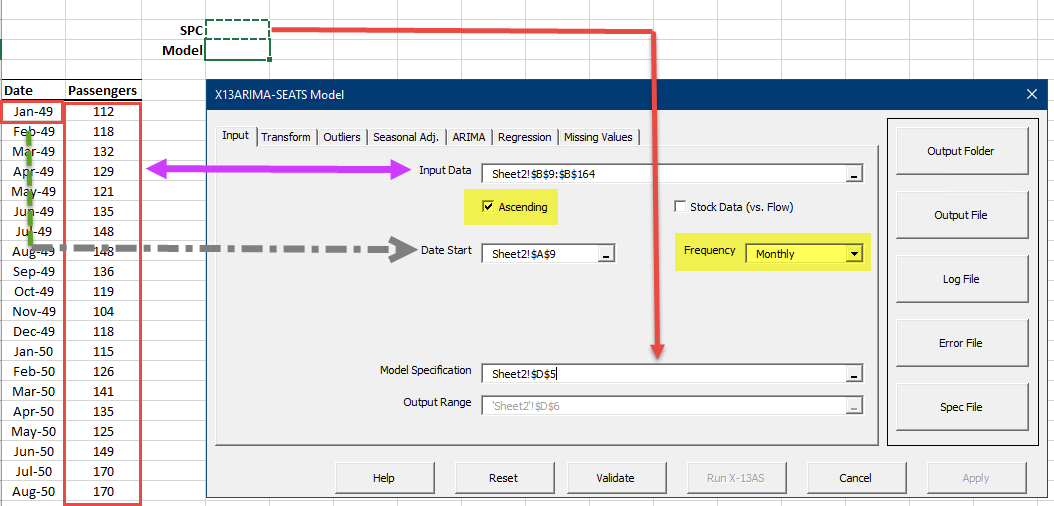4. In the X13AS window, the Input tab is active and displayed. We need to select and describe the input data set.
1. In the Input Data field, select the cells range in the worksheet of your data.
2. In the Date Start field, select the cell with the earliest date value.
3. The data set is collected every month, so no need to change the frequency field.
4. Select the empty cell above the currently selected cell to store the X13AS specification.
5. Select the Transform tab and click on Auto for the transform function. Leave everything on this tab unchanged.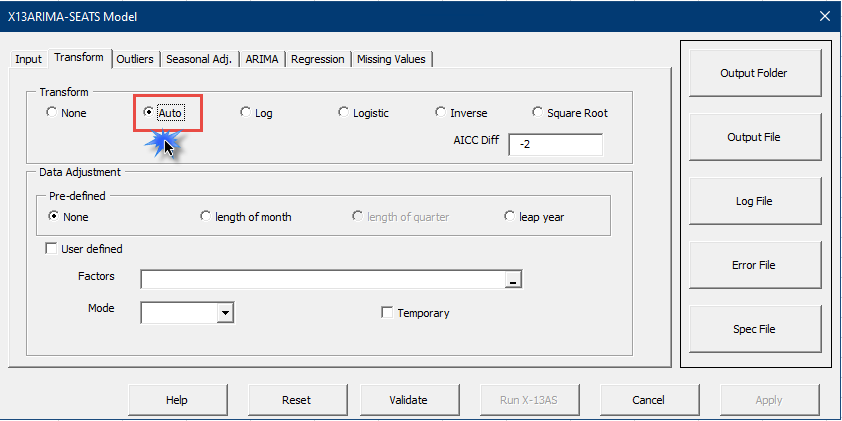6. Switch to the ARIMA tab, then:
1. The Auto (TRAMO) methodology is selected by default. Leave it.
2. Change the Number of Years in the Forecast section to 3 years.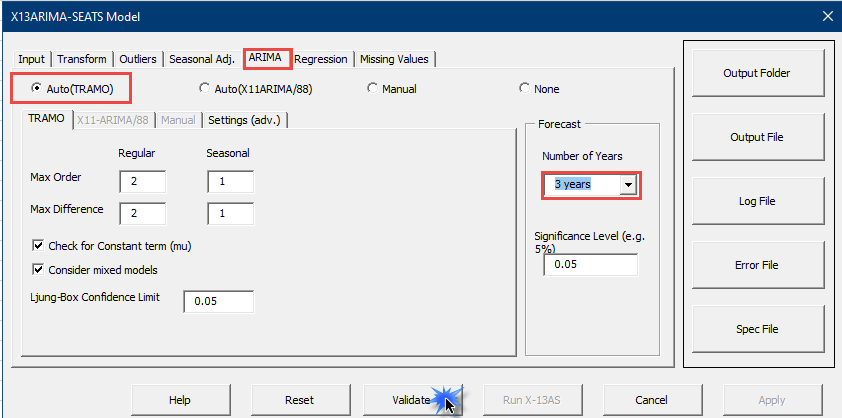7. Click the Validate command button. The Run X-13AS and Apply buttons are enabled now.
8. Next, click the Apply command button. The X13AS wizard will revise the formula and generate the model’s specifications into the value of the SPC cell.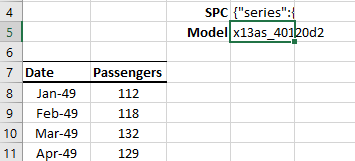## Step 3 – Query/Poll the Forecast Values

1. Now, we are ready to insert the forecast values of the X13AS model into our workbook. Create a header row for the output table in your workbook.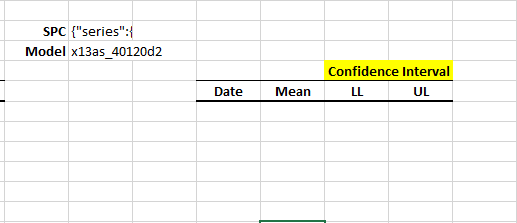2. Next, add the dates for future dates. The last observation date in the data set is Dec 1960, so the date in the forecast table should start on Jan 1961 and increase by one (1) month for each row.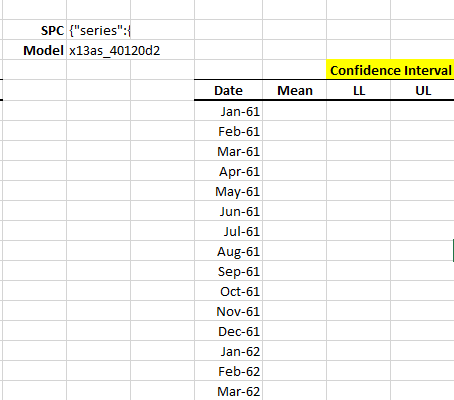3. Now we are ready to poll the forecast values from the X13AS model. To do so, type the X13ASFore(.) function in the formula toolbar.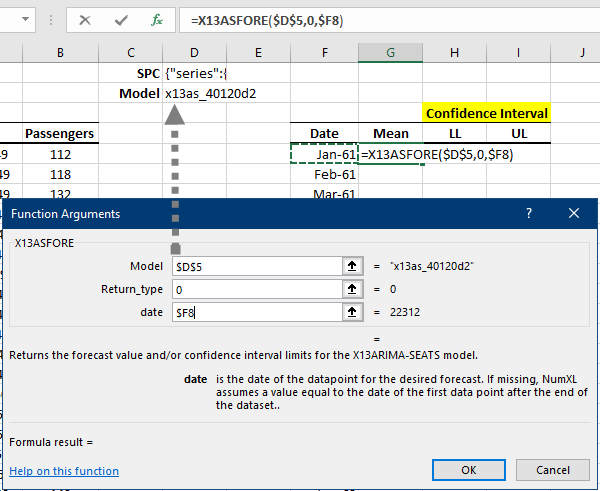4. Click OK. The mean forecast values for Jan 1961 will appear in our forecast table.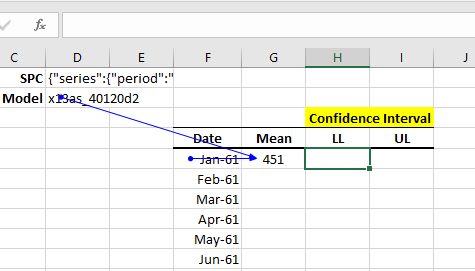5. Copy the formula to columns H and I, then edit the return_type argument to 1 and 2, respectively.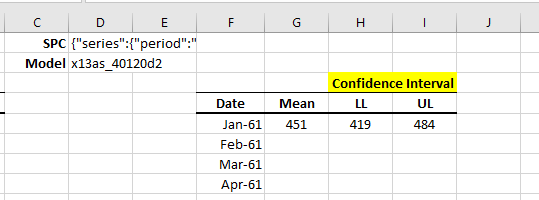6. Copy the first-row formulas to the remaining rows in the table.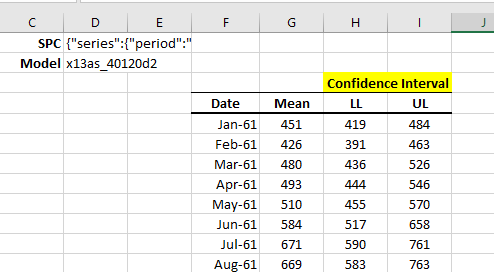7. Let’s now plot the forecast and its confidence interval in our spreadsheet.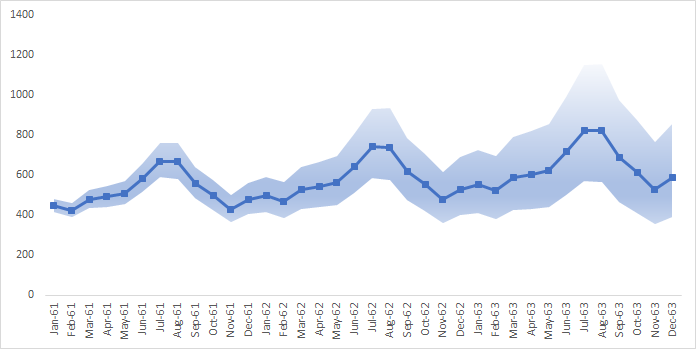Hint: To generate a similar graph in excel, do the following:

1. Calculate the difference between UL and LL in an adjacent column.
2. Plot the three series: mean forecast, lower limit, and the difference (UL-LL) on the same chart.
3. Change the chart type for each series as follows:
1. Forecast: Line with Markers
2. LL Series: Stacked Area
3. UL-LL (Difference): Stacked Area.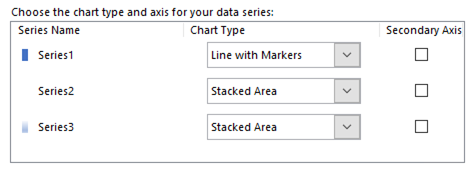4. Select the LL Series, edit the Format, and change the “Fill” property to No fill.